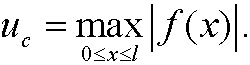# 7. Characteristic scales.

One important procedure while formulating a mathematical model of a physical situation is scaling. Roughly speaking, scaling deals with choosing new,  usually dimensionless variables and reformulate the problem expressed in these variables.

Example: Let t be the time. Every problem has a characteristic time tc, which is the smallest interval of time in which a notable change can be observed in the physical quantities. We then make the change of variables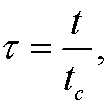to achieve a dimensionless time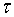.

Remark: We have different tc for different problems. If we study the motion of glaciers, tc can be of order years while tc can be of order microseconds if we for instance study nuclear reactions in atoms.

Example: (One dimensional heat conduction, see next part.) Consider a tall rod placed along the x-axis. The rod consists of a material with heat diffusivity k. We let u=u(x,t) denote the temperature in the point x at time t. The rod has the temperature 0 degrees at time t=0. We then keep the ends x=0 and x=l at the temperature T0 and examine how the temperature spreads in the rod. If the rod is long and slender, we can consider the problem one dimensional.The temperature distribution is given by the one dimensional heat equation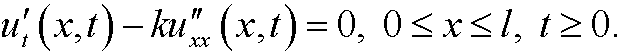We have the inital valueand the boundary conditions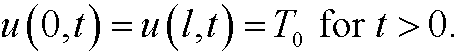As characteristic length lc we choose the length l of the rod and as characteristic temperature uc we choose the boundary temperature T0. As characteristic time tc we then choose l2/k, since k has the dimension [k]=L2/T. Thus we make the the change of variables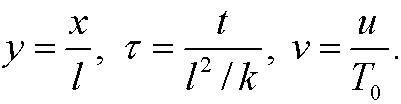The chain rule now gives thatThis implies that the heat equation is transformed tothe initial value to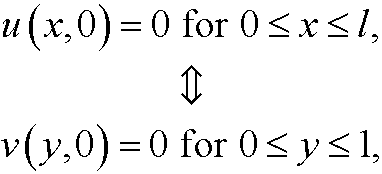and the boundary conditions toAlltogether, our transformed dimensionless boundary value problem will be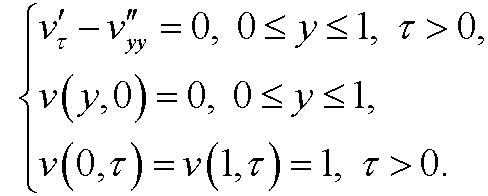If we solve the problem we see that the heat will distribute in the rod as in the figure below.Remark: If we changed the initial value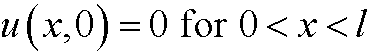toa natural choice of uc could be the mean valueHowever, it is more common and easier to choose